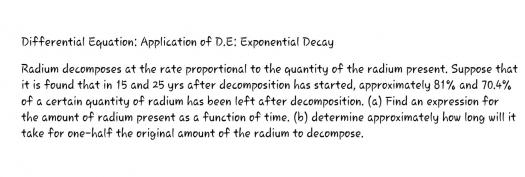# Differential Equation: Application of D.E: Exponential Decay### Differential Equation:

Differential Equation: Application of D.E: Exponential Decay

Radium decomposes at the rate proportional to the quantity of the radium present. Suppose that it is found that in 15 and 25 yrs after decomposition has started, approximately 81% and 70.4% of a certain quantity of radium has been left after decomposition. (a) Find an expression for the amount of radium present as a function of time. (b) determine approximately how long will it take for one-half the original amount of the radium to decompose.### Let x = amount of radium at

Let x = amount of radium at any time.
$\dfrac{dx}{dt} = kt$

$\dfrac{dx}{x} = k \, dt$

$\displaystyle \int \dfrac{dx}{x} = k \int dt$

$\ln x = kt + c$

When t = 15 yrs, x = 81%
$\ln 81 = 15k + c$   ←   (1)

When t = 25, x = 70.4%
$\ln 70.4 = 25k + c$   ←   (2)

From (1) and (2)
$k = -0.014$

$c = 4.605$

Hence,
$\ln x = -0.014t + 4.605$       answer for part (a)

$t = \dfrac{4.605 - \ln x}{0.014}$

For x = 50%
$t = \dfrac{4.605 - \ln 50}{0.014}$

$t = 49.5 ~ \text{years}$       answer for part (b)### Another solution (By

Another solution (By Calculator - CASIO fx-991ES PLUS):
MODE 3 5

X Y
15 81
25 70.4

AC
$t = 50\hat{x} = 59.4 ~ \text{years}$

Note:
$\hat{x}$ = SHIFT 1 5 4

• Mathematics inside the configured delimiters is rendered by MathJax. The default math delimiters are $$...$$ and $...$ for displayed mathematics, and $...$ and $...$ for in-line mathematics.